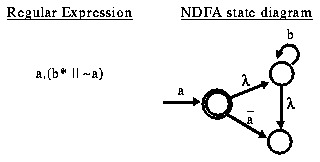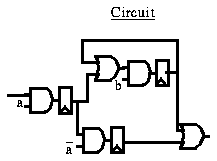## An Example Regular Expression and NDFA

Classical regular expressions have three operators, and a alphabet of input symbols (identifiers). The three operators are concatenation, Kleene closure, and or. Concatenation describes two inputs occuring in sequence, and in PBS is denoted by "," (comma). Kleene closure describes zero or more occurences of an input, and is represented by "*" (asterisk). The third classical operator, or, describes the logical or between to inputs. These operators can be combined to describe any kind of input sequence. For example:The above regular expression has three identifiers (which represent arbitrary logic functions of the primary input), "a", "b", and "~a". The corresponding NDFA state diagram has three states. The circuit implementation is shown below, and uses five gates and three memory elements to recognize the regular expression. A circuit implementation can be constructed from the NDFA or directly from the regular expression, and requires at most one memory element per NDFA state or regular expression identifier.` `